# Histogram & Scatterplot in R - using mtcars dataset

A scatter plot is a useful way to visualize two quantitative variables in a dataset.

We are exploring mtcars dataset for some amazing data visualization.

We check mtcars dataset description by using following code:

?mtcars

It will open mtcars dataset description in Help window

Contact at TJT@TechnicalJockey.com , if you are looking for an Instructor Based Online Training !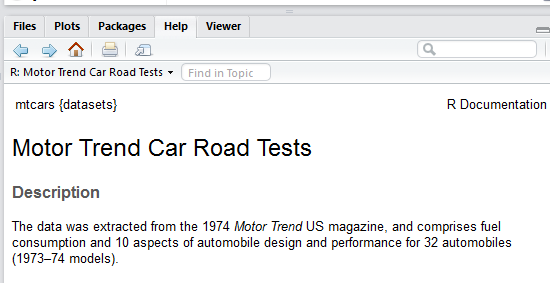We attached mtcars dataset in R. By attaching dataset ,we can use variables directly of mtcars .

attach(mtcars)

?plot

For plot graph between any variables , we use plot() .

plot(wt,mpg)

It will plot between mpg and wt in Plots window.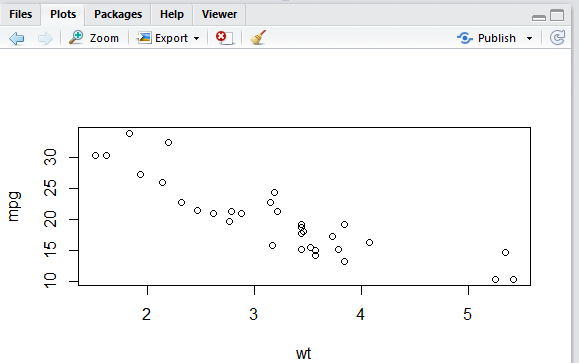We can add labels associated with axis and color by using this code :

plot(wt,mpg,col="red",ylab="Miles/(US) gallon",xlab="Weight (1000 lbs)")

We add label in x-axis by using xlab parameter and label in y-axis by using ylab parameter.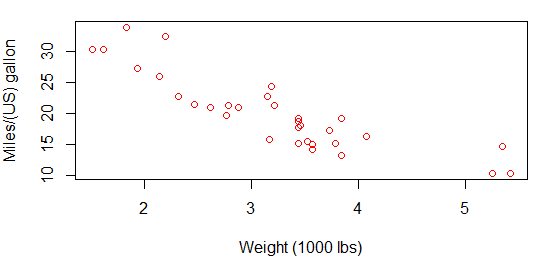We can add naming of graph by using main parameter .

plot(wt,mpg,col="red",ylab="Miles/(US) gallon",xlab="Weight (1000 lbs)",main ="Miles per Gallon and Weight")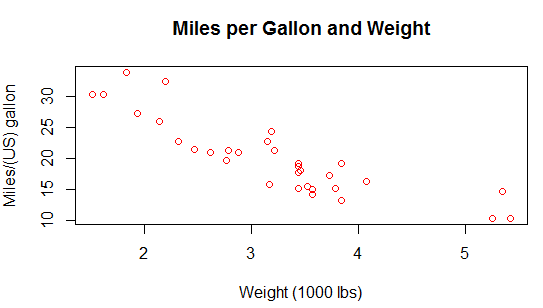hist()

It is used to plot histogram . It is an estimate of the probability distribution of a continuous variable.

hist(mpg,col="red")

It shows red frequency bars of mpg variable.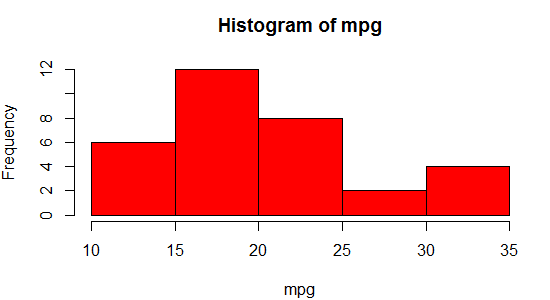We add breaks parameter , it is used to break the x-axis in given number of breaks.

hist(mpg,col="red",breaks = 10)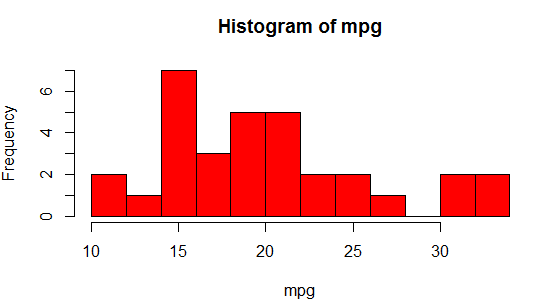We assign freq parameter to FALSE to draw lines in density plot.

We can also density plot by using this code:

hist(mpg,col="red",freq = FALSE)

lines(density(mpg))

We are using ggplot2 package for more advanced visualization.

We install ggplot2 package :

install.packages("ggplot2")

library(ggplot2)

We are using qplot to plot graphs.

?qplot

We determine dimensions of mtcars. It contains 32 rows and 11 columns.

dim(mtcars)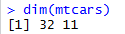We can see top 6 observations of mtcars dataset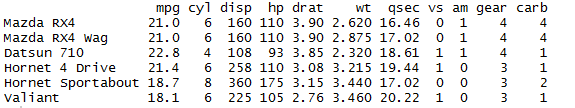We are using qplot() function to plot scatter plot between mpg and wt .

qplot(mpg, wt, data = mtcars)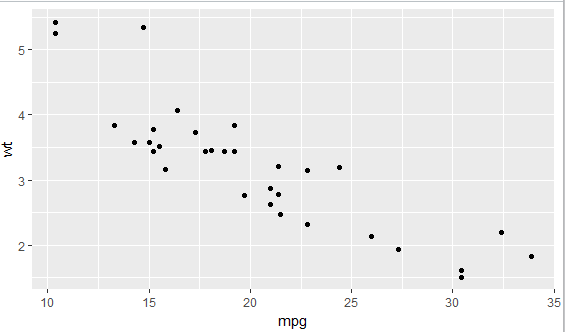We have cyl variable which shows number of cylinders. We add colors by cyl values as:

qplot(mpg, wt, data = mtcars, col= cyl)We change cyl to factor . So , color select values on the basis of cyl value.

qplot(mpg, wt, data = mtcars, colour =  factor(cyl))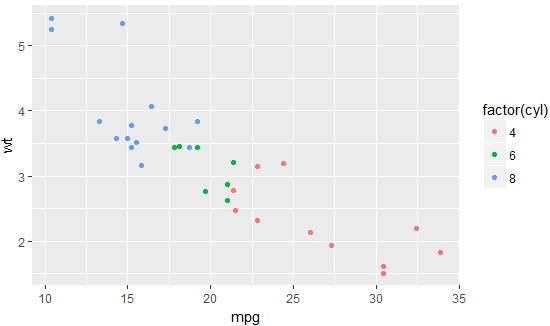We add size and shape according to cyl values .

qplot(mpg, wt, data = mtcars,shape=factor(cyl), size = factor(cyl),  colour =  factor(cyl))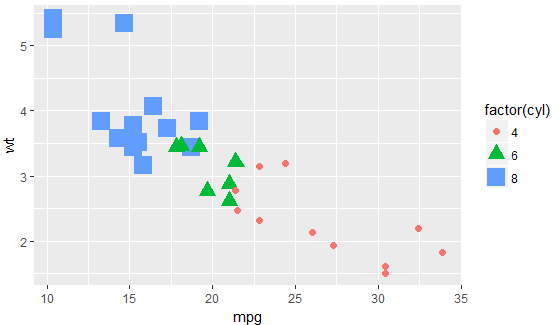We added two variables by using facets parameter. It is used to add formula to add variables.

qplot(mpg, wt, data = mtcars, facets = vs ~ am)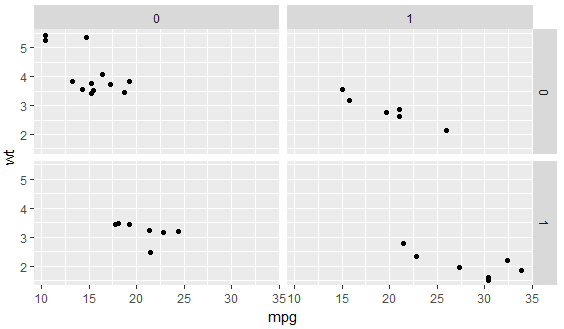Similarly , we add formula in facets parameter to represent am and cyl variables.

qplot(mpg, wt, data = mtcars, facets = am ~ cyl)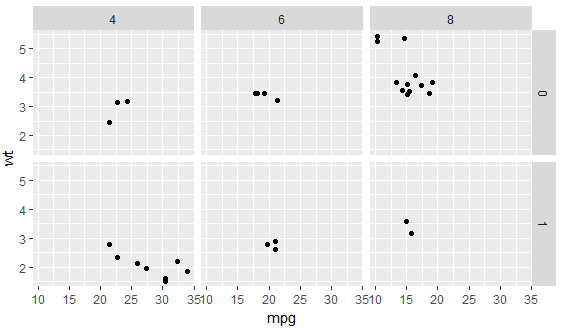qplot(mpg,wt,data=mtcars,facets=cyl~.,colour=factor(cyl))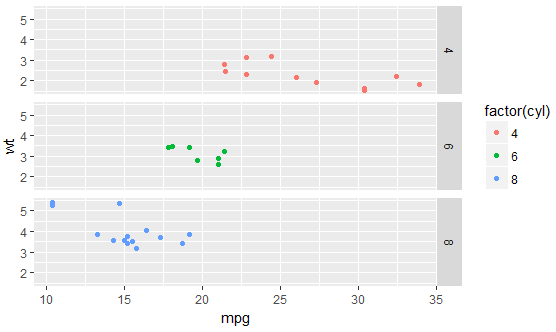Contact at TJT@TechnicalJockey.com , if you are looking for an Instructor Based Online Training !

There are no entries yet.## Looking for Corporate Training ? Reach out to us at Akriti.Lal@instrovate.com

Reach out to us if you are looking for Corporate Training to Build The Next Generation Analytical Workforce with an in-depth understanding of  Exploratory Data Analysis , Data Visualisation, Data Analytics , AI First , Machine Learning & Deep Learning Training & Consulting helping them to take Data Informed Decision at each stage of the business.

We understand that At the present times , the Entire Industry is in a Tranformation stage with the Softwares  being rebuilt with Artificial Intelligence Capabilities .

We need SMART WORKFORCE for the SMART SOFTWARES to reap the maximum return .

Whatsapp at +91-9953805788 or email at - akriti.lal@instrovate.com if you would like to know more .

• Corporate Tableau Training in Gurgaon
• Corporate Data Analytics Training in Gurgaon
• Corporate Microsoft Power BI Training in Gurgaon
• Corporate Microstrategy Training in Gurgaon
• Corporate Google Data Studio Training in Gurgaon
• Corporate Python Training in Gurgaon
• Corporate Advance Analytics in R Programming Training in Gurgaon
• Corporate Machine Learning Training in Gurgaon
• Corporate Deep Learning Training in Gurgaon
• Corporate Data Visualization Training in Gurgaon

Instrovate Technologies

Noida, Gurgaon

akriti.lal@instrovate.com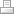Print | Sitemap